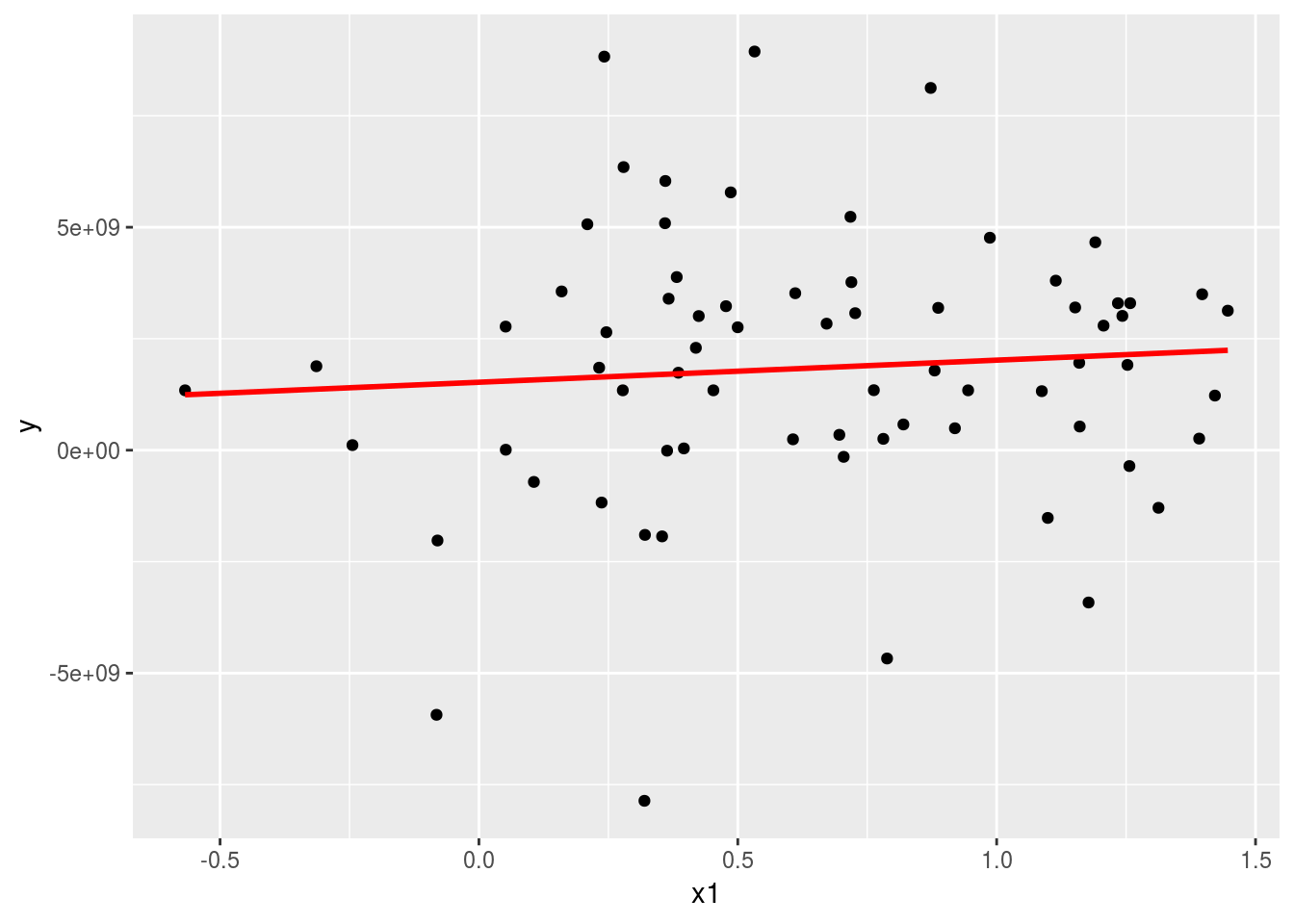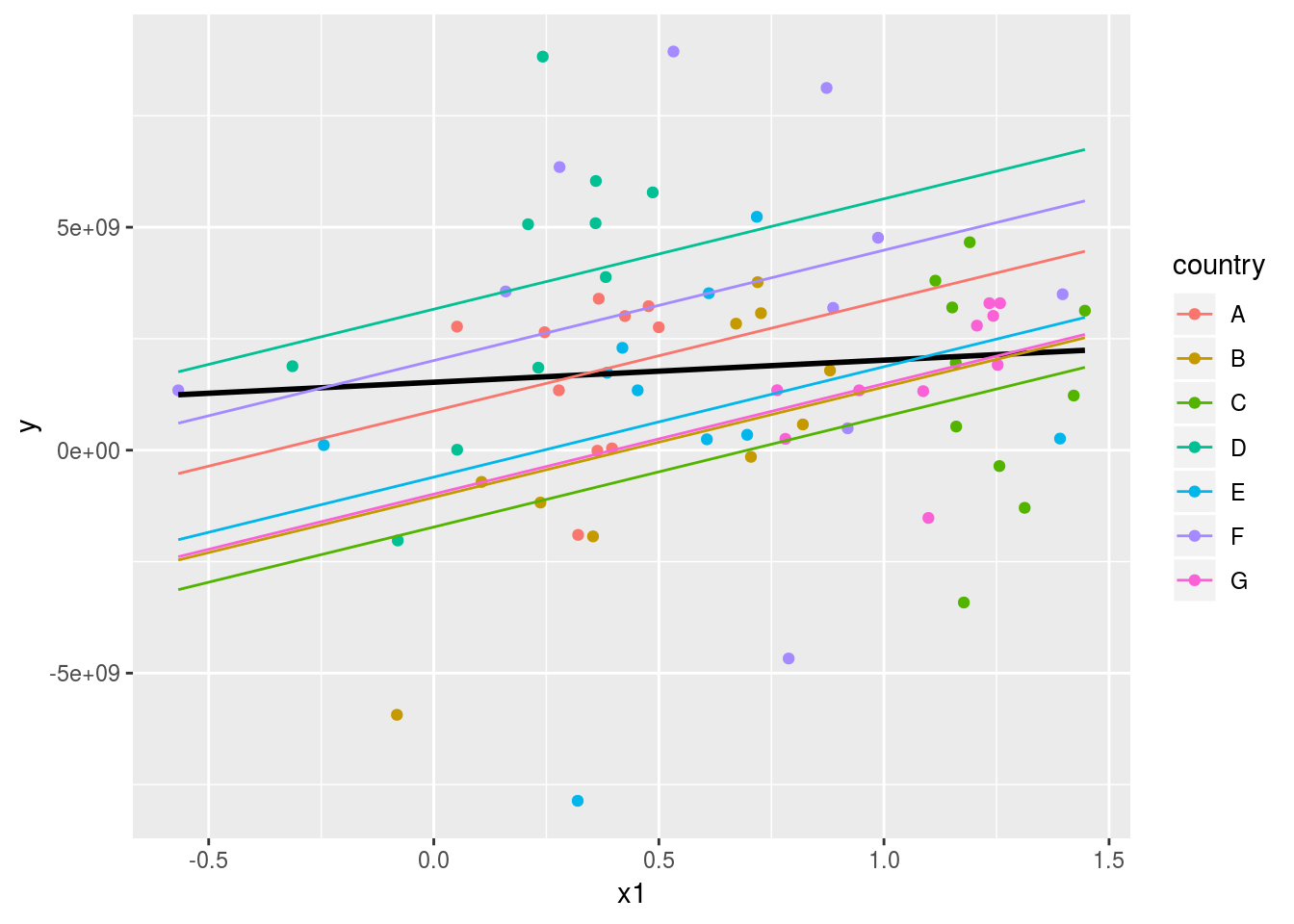# Chapter 6 Fixed or random effects

This section was originally prepared for the Adanced Methods of Political Analysis (Poli 706) in Spring 2019, which I served as a TA for Tobias Heinrich. The goal of this part is to address one common question we encounter in our research: when to use fixed or random effects?

## 6.1 Prerequisite

Load the following packages for this section.

library(modelr)
library(tidyverse)
library(foreign)
library(plm)

## 6.2 Introduction

Let us write down the following model (from Wooldridge p.435).

$$y_{it}=\beta_{1}x_{it} + a_{i} + u_{it}$$

Essentially, we have some “fixed” errors ($$a_{i}$$) over time. When we add the additional assumption that $$a_{i}$$ is uncorrelated with any explanatory variable, we have the random effects model.

Let us get started by using the following data to run a simple OLS.

Panel <- read.dta("http://dss.princeton.edu/training/Panel101.dta")
ols <-lm(y ~ x1, data=Panel)

Now plot the fitted line.

grid <- data.frame(Intercept=1, x1=seq_range(Panel$x1, 10)) grid$pred <- predict(ols,grid)
ggplot(Panel, aes(x1)) +
geom_point(aes(y = y)) +
geom_line(aes(y = pred), data = grid, colour = "red", size = 1)## 6.3 Fixed effects

There are two common ways to fit fixed effects. The first one is to addd dummy variables for the group indicator.

fixed_dum <-lm(y ~ x1 + factor(country) - 1, data=Panel)
summary(fixed_dum)
##
## Call:
## lm(formula = y ~ x1 + factor(country) - 1, data = Panel)
##
## Residuals:
##        Min         1Q     Median         3Q        Max
## -8.634e+09 -9.697e+08  5.405e+08  1.386e+09  5.612e+09
##
## Coefficients:
##                    Estimate Std. Error t value Pr(>|t|)
## x1                2.476e+09  1.107e+09   2.237  0.02889 *
## factor(country)A  8.805e+08  9.618e+08   0.916  0.36347
## factor(country)B -1.058e+09  1.051e+09  -1.006  0.31811
## factor(country)C -1.723e+09  1.632e+09  -1.056  0.29508
## factor(country)D  3.163e+09  9.095e+08   3.478  0.00093 ***
## factor(country)E -6.026e+08  1.064e+09  -0.566  0.57329
## factor(country)F  2.011e+09  1.123e+09   1.791  0.07821 .
## factor(country)G -9.847e+08  1.493e+09  -0.660  0.51190
## ---
## Signif. codes:  0 '***' 0.001 '**' 0.01 '*' 0.05 '.' 0.1 ' ' 1
##
## Residual standard error: 2.796e+09 on 62 degrees of freedom
## Multiple R-squared:  0.4402, Adjusted R-squared:  0.368
## F-statistic: 6.095 on 8 and 62 DF,  p-value: 8.892e-06
grid_fixdum <- expand(data.frame(Intercept=1, x1=seq_range(Panel$x1, 14), country=unique(Panel$country)),
x1, country)
grid_fixdum\$pred <- predict(fixed_dum,grid_fixdum)
ggplot(Panel, aes(x1)) +
geom_point(aes(y = y, colour=country)) +
geom_smooth(aes(y = y), method='lm', se=FALSE, colour="black")+
geom_line(data=grid_fixdum, aes(x=x1, y=pred, colour=country))The second way is to incorporate fixed effects directly. In R, it is typically referred to as “within” model.

fixed <- plm(y ~ x1, data=Panel, index=c("country", "year"), model="within")
summary(fixed)
## Oneway (individual) effect Within Model
##
## Call:
## plm(formula = y ~ x1, data = Panel, model = "within", index = c("country",
##     "year"))
##
## Balanced Panel: n = 7, T = 10, N = 70
##
## Residuals:
##      Min.   1st Qu.    Median      Mean   3rd Qu.      Max.
## -8.63e+09 -9.70e+08  5.40e+08  0.00e+00  1.39e+09  5.61e+09
##
## Coefficients:
##      Estimate Std. Error t-value Pr(>|t|)
## x1 2475617827 1106675594   2.237  0.02889 *
## ---
## Signif. codes:  0 '***' 0.001 '**' 0.01 '*' 0.05 '.' 0.1 ' ' 1
##
## Total Sum of Squares:    5.2364e+20
## Residual Sum of Squares: 4.8454e+20
## R-Squared:      0.074684
## Adj. R-Squared: -0.029788
## F-statistic: 5.00411 on 1 and 62 DF, p-value: 0.028892

We can then extract the fixed effects.

fixef(fixed) 
##           A           B           C           D           E           F
##   880542404 -1057858363 -1722810755  3162826897  -602622000  2010731793
##           G
##  -984717493

We can also test whether the fixed effects model is better than OLS.

pFtest(fixed, ols) 
##
##  F test for individual effects
##
## data:  y ~ x1
## F = 2.9655, df1 = 6, df2 = 62, p-value = 0.01307
## alternative hypothesis: significant effects

## 6.4 Random effects

random <- plm(y ~ x1, data=Panel, index=c("country", "year"), model="random")
summary(random)
## Oneway (individual) effect Random Effect Model
##    (Swamy-Arora's transformation)
##
## Call:
## plm(formula = y ~ x1, data = Panel, model = "random", index = c("country",
##     "year"))
##
## Balanced Panel: n = 7, T = 10, N = 70
##
## Effects:
##                     var   std.dev share
## idiosyncratic 7.815e+18 2.796e+09 0.873
## individual    1.133e+18 1.065e+09 0.127
## theta: 0.3611
##
## Residuals:
##      Min.   1st Qu.    Median      Mean   3rd Qu.      Max.
## -8.94e+09 -1.51e+09  2.82e+08  0.00e+00  1.56e+09  6.63e+09
##
## Coefficients:
##               Estimate Std. Error z-value Pr(>|z|)
## (Intercept) 1037014284  790626206  1.3116   0.1896
## x1          1247001782  902145601  1.3823   0.1669
##
## Total Sum of Squares:    5.6595e+20
## Residual Sum of Squares: 5.5048e+20
## R-Squared:      0.02733
## Adj. R-Squared: 0.013026
## Chisq: 1.91065 on 1 DF, p-value: 0.16689

### 6.4.1 Fixed or random

You can run a Hausman test (which tests whether the unique errors are correlated with the regressors, the null is they are not). If the p-value is significant, then you choose fixed effects (since the unique errors are correlated with the regressors).

phtest(fixed, random)
##
##  Hausman Test
##
## data:  y ~ x1
## chisq = 3.674, df = 1, p-value = 0.05527
## alternative hypothesis: one model is inconsistent

## 6.5 More tests

You can also add time fixed effects.

fixed_time_dum <- plm(y ~ x1 + factor(year), data=Panel, index=c("country", "year"), model="within")
summary(fixed_time_dum)
## Oneway (individual) effect Within Model
##
## Call:
## plm(formula = y ~ x1 + factor(year), data = Panel, model = "within",
##     index = c("country", "year"))
##
## Balanced Panel: n = 7, T = 10, N = 70
##
## Residuals:
##      Min.   1st Qu.    Median      Mean   3rd Qu.      Max.
## -7.92e+09 -1.05e+09 -1.40e+08  0.00e+00  1.63e+09  5.49e+09
##
## Coefficients:
##                    Estimate Std. Error t-value Pr(>|t|)
## x1               1389050354 1319849567  1.0524  0.29738
## factor(year)1991  296381558 1503368528  0.1971  0.84447
## factor(year)1992  145369666 1547226548  0.0940  0.92550
## factor(year)1993 2874386795 1503862554  1.9113  0.06138 .
## factor(year)1994 2848156288 1661498927  1.7142  0.09233 .
## factor(year)1995  973941306 1567245748  0.6214  0.53698
## factor(year)1996 1672812557 1631539254  1.0253  0.30988
## factor(year)1997 2991770063 1627062032  1.8388  0.07156 .
## factor(year)1998  367463593 1587924445  0.2314  0.81789
## factor(year)1999 1258751933 1512397632  0.8323  0.40898
## ---
## Signif. codes:  0 '***' 0.001 '**' 0.01 '*' 0.05 '.' 0.1 ' ' 1
##
## Total Sum of Squares:    5.2364e+20
## Residual Sum of Squares: 4.0201e+20
## R-Squared:      0.23229
## Adj. R-Squared: 0.00052851
## F-statistic: 1.60365 on 10 and 53 DF, p-value: 0.13113

Or simply,

fixed_time <- plm(y ~ x1, data=Panel, index=c("country", "year"), model="within", effect="twoway")
summary(fixed_time)
## Twoways effects Within Model
##
## Call:
## plm(formula = y ~ x1, data = Panel, effect = "twoway", model = "within",
##     index = c("country", "year"))
##
## Balanced Panel: n = 7, T = 10, N = 70
##
## Residuals:
##      Min.   1st Qu.    Median      Mean   3rd Qu.      Max.
## -7.92e+09 -1.05e+09 -1.40e+08  0.00e+00  1.63e+09  5.49e+09
##
## Coefficients:
##      Estimate Std. Error t-value Pr(>|t|)
## x1 1389050354 1319849567  1.0524   0.2974
##
## Total Sum of Squares:    4.1041e+20
## Residual Sum of Squares: 4.0201e+20
## R-Squared:      0.020471
## Adj. R-Squared: -0.27524
## F-statistic: 1.10761 on 1 and 53 DF, p-value: 0.29738

### 6.5.1 Test whether adding time-fixed effects is necessary

pFtest(fixed_time, fixed)
##
##  F test for twoways effects
##
## data:  y ~ x1
## F = 1.209, df1 = 9, df2 = 53, p-value = 0.3094
## alternative hypothesis: significant effects